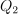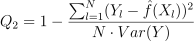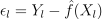# MetaModelValidation¶

class MetaModelValidation(*args)

Base class to score a metamodel and perform validations.

Parameters:
inputValidationSample, outputValidationSample2-d sequence of float

The input and output validation samples, not used during the learning step.

metaModelFunction

Metamodel to validate.

Notes

A MetaModelValidation object is used for the validation process of a metamodel. For that purpose, a dataset independent of the learning step, is used to score the surrogate model. Its main functionalities are :

• To compute the predictivity factor• To get the residual sample, its non parametric distribution

• To draw a model vs metamodel validation graph.

Currently only one dimensional output models are available.

Examples

>>> import openturns as ot
>>> from math import pi
>>> dist = ot.Uniform(-pi/2, pi/2)
>>> # Model here is sin(x)
>>> model = ot.SymbolicFunction(['x'], ['sin(x)'])
>>> # We can build several types of models (kriging, pc, ...)
>>> # We use a Taylor development (order 5) and compare the metamodel with the model
>>> metaModel = ot.SymbolicFunction(['x'], ['x - x^3/6.0 + x^5/120.0'])
>>> x = dist.getSample(10)
>>> y = model(x)
>>> # Validation of the model
>>> val = ot.MetaModelValidation(x, y, metaModel)
>>> # Compute the first indicator : q2
>>> q2 = val.computePredictivityFactor()
>>> # Get the residual
>>> residual = val.getResidualSample()
>>> # Get the histogram of residual
>>> histoResidual = val.getResidualDistribution(False)
>>> # Draw the validation graph
>>> graph = val.drawValidation()


Methods

 Compute the predictivity factor. Plot a model vs metamodel graph for visual validation. Accessor to the object's name. Accessor to the object's id. Accessor to the input sample. Accessor to the object's name. Accessor to the output sample. getResidualDistribution([smooth]) Compute the non parametric distribution of the residual sample. Compute the residual sample. Accessor to the object's shadowed id. Accessor to the object's visibility state. Test if the object is named. Test if the object has a distinguishable name. setName(name) Accessor to the object's name. Accessor to the object's shadowed id. setVisibility(visible) Accessor to the object's visibility state.
__init__(*args)
computePredictivityFactor()

Compute the predictivity factor.

Returns:
q2Point

The predictivity factor

Notes

The predictivity factoris given by :drawValidation()

Plot a model vs metamodel graph for visual validation.

Returns:
graphGridLayout

The visual validation graph.

getClassName()

Accessor to the object’s name.

Returns:
class_namestr

The object class name (object.__class__.__name__).

getId()

Accessor to the object’s id.

Returns:
idint

Internal unique identifier.

getInputSample()

Accessor to the input sample.

Returns:
inputSampleSample

Input sample of a model evaluated apart.

getName()

Accessor to the object’s name.

Returns:
namestr

The name of the object.

getOutputSample()

Accessor to the output sample.

Returns:
outputSampleSample

Output sample of a model evaluated apart.

getResidualDistribution(smooth=True)

Compute the non parametric distribution of the residual sample.

Parameters:
smoothbool

Tells if distribution is smooth (true) or not. Default argument is true.

Returns:
residualDistributionDistribution

The residual distribution.

Notes

The residual distribution is built thanks to KernelSmoothing if smooth argument is true. Otherwise, an histogram distribution is returned, thanks to HistogramFactory.

getResidualSample()

Compute the residual sample.

Returns:
residualSample

The residual sample.

Notes

The residual sample is given by :Accessor to the object’s shadowed id.

Returns:
idint

Internal unique identifier.

getVisibility()

Accessor to the object’s visibility state.

Returns:
visiblebool

Visibility flag.

hasName()

Test if the object is named.

Returns:
hasNamebool

True if the name is not empty.

hasVisibleName()

Test if the object has a distinguishable name.

Returns:
hasVisibleNamebool

True if the name is not empty and not the default one.

setName(name)

Accessor to the object’s name.

Parameters:
namestr

The name of the object.

Accessor to the object’s shadowed id.

Parameters:
idint

Internal unique identifier.

setVisibility(visible)

Accessor to the object’s visibility state.

Parameters:
visiblebool

Visibility flag.

## Examples using the class¶Validate a polynomial chaos

Validate a polynomial chaosCreate a polynomial chaos metamodel by integration on the cantilever beam

Create a polynomial chaos metamodel by integration on the cantilever beamCreate a polynomial chaos metamodel

Create a polynomial chaos metamodelCreate a polynomial chaos for the Ishigami function: a quick start guide to polynomial chaos

Create a polynomial chaos for the Ishigami function: a quick start guide to polynomial chaosChaos cross-validation

Chaos cross-validationPolynomial chaos is sensitive to the degree

Polynomial chaos is sensitive to the degreeCreate a sparse chaos by integration

Create a sparse chaos by integrationCompute Sobol’ indices confidence intervals

Compute Sobol' indices confidence intervalsKriging : cantilever beam model

Kriging : cantilever beam modelKriging the cantilever beam model using HMAT

Kriging the cantilever beam model using HMATExample of multi output Kriging on the fire satellite model

Example of multi output Kriging on the fire satellite modelChoose the trend basis of a kriging metamodel

Choose the trend basis of a kriging metamodelSequentially adding new points to a kriging

Sequentially adding new points to a kriging